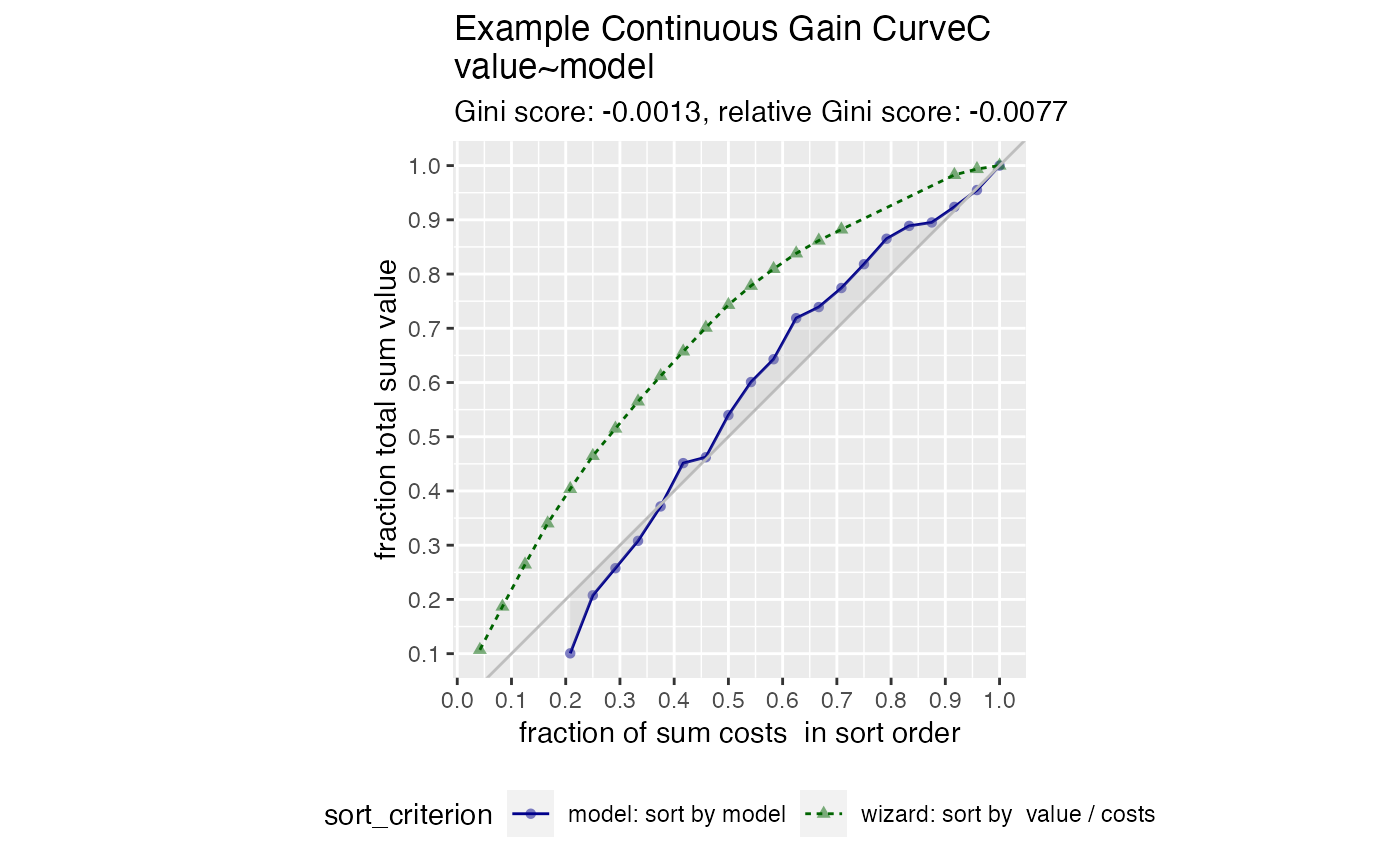Plot the cumulative gain curve of a sort-order with costs.

GainCurvePlotC(
frame,
xvar,
costVar,
truthVar,
title,
...,
estimate_sig = FALSE,
large_count = 1000,
model_color = "darkblue",
wizard_color = "darkgreen",
)

## Arguments

frame data frame to get values from name of the independent (input or model score) column in frame cost of each item (drives x-axis sum) name of the dependent (output or result to be modeled) column in frame title to place on plot no unnamed argument, added to force named binding of later arguments. logical, if TRUE compute significance numeric, upper bound target for number of plotting points color for the model curve color for the "wizard" (best possible) curve color for the shaded area under the curve

## Details

GainCurvePlotC plots a cumulative gain curve for the case where items have an additional cost, in addition to an outcome value.

The x-axis represents the fraction of total cost experienced when items are sorted by score, and the y-axis represents the cumulative summed true outcome represented by the items seen so far.

For comparison, GainCurvePlotC also plots the "wizard curve": the gain curve when the data is sorted according to its true outcome/cost (the optimal sort order).

To improve presentation quality, the plot is limited to approximately large_count points (default: 1000). For larger data sets, the data is appropriately randomly sampled down before plotting.

GainCurvePlot

## Examples


set.seed(34903490)
y = abs(rnorm(20)) + 0.1
x = abs(y + 0.5*rnorm(20))
frm = data.frame(model=x, value=y)
frm$costs=1 frm$costs=5
WVPlots::GainCurvePlotC(frm, "model", "costs", "value",
title="Example Continuous Gain CurveC")